﻿ 基于磁流变弹性体基础隔振的电力变压器可控减振研究 Research on Controllable Vibration Reduction of Power Transformer with Magnetorheological Elastic Foundation

Journal of Electrical Engineering
Vol. 08  No. 04 ( 2020 ), Article ID: 39498 , 7 pages
10.12677/JEE.2020.84020

1江苏科能电力工程咨询有限公司，江苏 南京

2南京师范大学电气与自动化工程学院，江苏 南京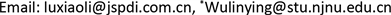Research on Controllable Vibration Reduction of Power Transformer with Magnetorheological Elastic Foundation

Wenjing Wang1, Xiaoli Lu1, Dong Lu1, Zhongxi Liu1, Bin Yu1, Danhui Kong1, Linying Wu2*

1Jiangsu Keneng Electric Power Engineering Consulting Co. LTD, Nanjing Jiangsu

2School of Electrical and Automation Engineering, Nanjing Normal University, Nanjing JiangsuReceived: Nov. 24th, 2020; accepted: Dec. 22nd, 2020; published: Dec. 29th, 2020ABSTRACT

The power transformer is sustained vibrating in operation, which will not only produce noise and affect the daily life of residents, but also seriously reduce the service life of power equipment and endanger the safety of the power grid. In this paper, a novel basic vibration isolation of magnetorheological elastomer is used for the power transformers, and the three-stage vibration reduction mode is realized by using the adjustable rigidity characteristics of magnetorheological elastomers to realize a controllable damping system applied to power transformers. The dynamic model of the system is further established. The comprehensive performance of the three-stage vibration reduction mode is analyzed under three excitations of harmonic, impact and random excitation. The results show that adjusting the stiffness of magnetorheological elastomer can effectively change the resonance frequency of power transformers under different external excitations. This work lays the foundation for further development of controller design.

Keywords:Power Transformer, Magnetorheological Elastomer, Controllable Vibration Reduction System, Three-Stage Vibration Reduction1. 引言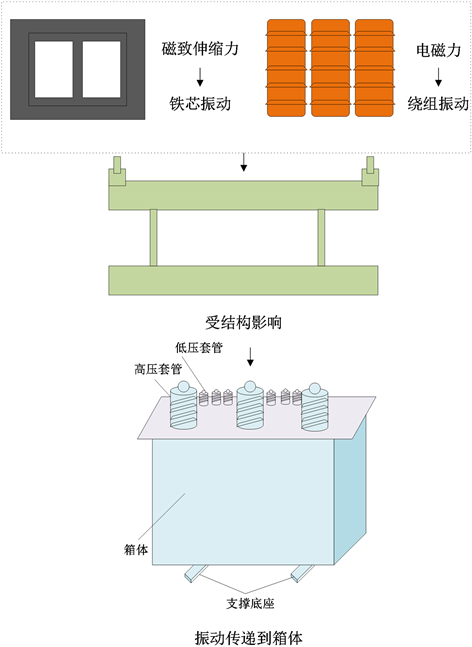Figure 1. Schematic diagram of transformer vibration transfer

2. 基于MRE的电力变压器主动减振系统

${m}_{1}{\stackrel{¨}{x}}_{1}+{c}_{1}\left({\stackrel{˙}{x}}_{1}-{\stackrel{˙}{x}}_{2}\right)+{k}_{1}\left({x}_{1}-{x}_{2}\right)=F\left(t\right)$ (1)

${m}_{2}{\stackrel{¨}{x}}_{2}-{c}_{1}\left({\stackrel{˙}{x}}_{1}-{\stackrel{˙}{x}}_{2}\right)-{k}_{1}\left({x}_{1}-{x}_{2}\right)+{k}_{2}{x}_{2}=0$ (2)

$F={k}_{1}x+{c}_{1}\stackrel{˙}{x}$ (3)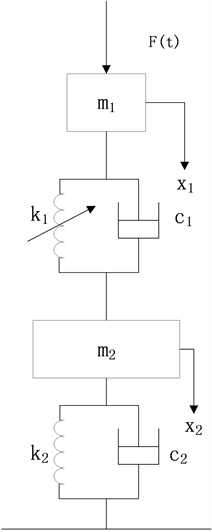Figure 2. Dynamic model of active vibration reduction system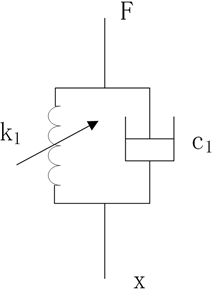Figure 3. MRE dynamic model

3. 仿真分析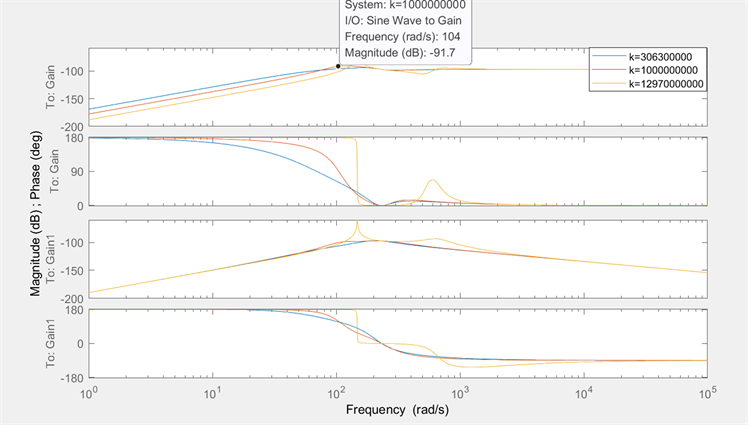Figure 4. The transmission rate of system vibration under harmonic excitation

$\left\{\begin{array}{l}{x}_{i1,2}=0.25{a}_{m}{\text{e}}^{2}{\left(\mu {\omega }_{0}t\right)}^{2}{\text{e}}^{-\mu {\omega }_{0}t}\\ {x}_{i3,4}=0.25{a}_{m}{\text{e}}^{2}{\left[\mu {\omega }_{0}\left(t-{t}_{0}\right)\right]}^{2}{\text{e}}^{-\mu {\omega }_{0}\left(t-{t}_{0}\right)}u\left(t-{t}_{0}\right)\end{array}$ (4)

${x}_{i}=A\mathrm{sin}\left(2\text{π}ft\right)$ (5)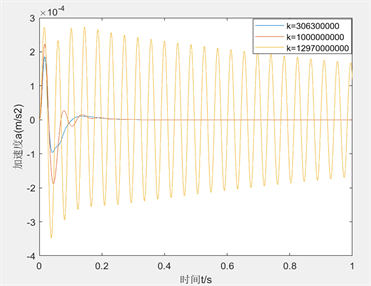Figure 5. Contrast diagram of acceleration under shock excitation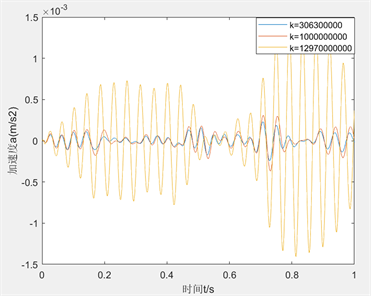Figure 6. Comparison diagram of acceleration under random excitation

4. 结论

Research on Controllable Vibration Reduction of Power Transformer with Magnetorheological Elastic Foundation[J]. 电气工程, 2020, 08(04): 179-185. https://doi.org/10.12677/JEE.2020.84020

1. 1. 胡行淼. 浅谈电力工程发展现状与未来趋势[J]. 建材与装饰, 2018(34): 231.

2. 2. 王中煜, 姚雁南. 试析电力技术发展与电力生产安全[J]. 中外企业家, 2018(18): 125.

3. 3. 侯东, 段炼, 曹春诚, 邴龙, 马超群, 陈鹤. 35 kV油浸式配电变压器噪声与振动测试[J]. 应用声学, 2020, 39(6): 964-968.

4. 4. 李诚理. 配电房干式变压器噪声分析及减振降噪处理[J]. 机电信息, 2020(2): 70-71.

5. 5. 顾鸣伟, 仝旭, 何守磊, 成果, 殷振. 变压器弹簧减振降噪装置的设计研究[J]. 机械工程与自动化, 2018(5): 116-118.

6. 6. 郭咏雪, 何立东, 李宽. 基于磁流变阻尼器的密封减振技术实验研究[J]. 振动与冲击, 2019, 38(22): 144-148.

7. 7. 袁杰, 陈磊. 磁流变液减振技术现状及方法研究[J]. 机械研究与应用, 2013(6): 195-198.

8. 8. 侯锁军, 黄一鸣, 史文库. 磁流变减振器对军用越野车平顺性及操稳性的影响[J]. 机械设计与制造, 2020(6): 146-152.